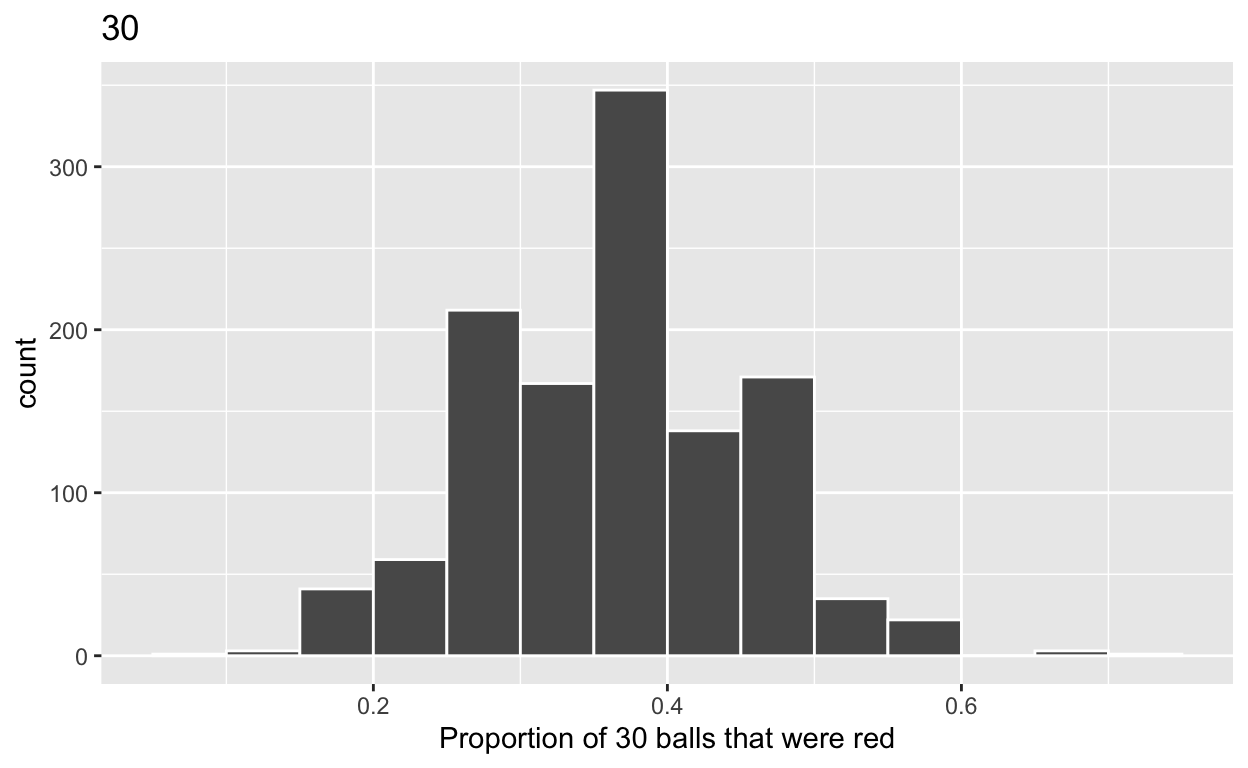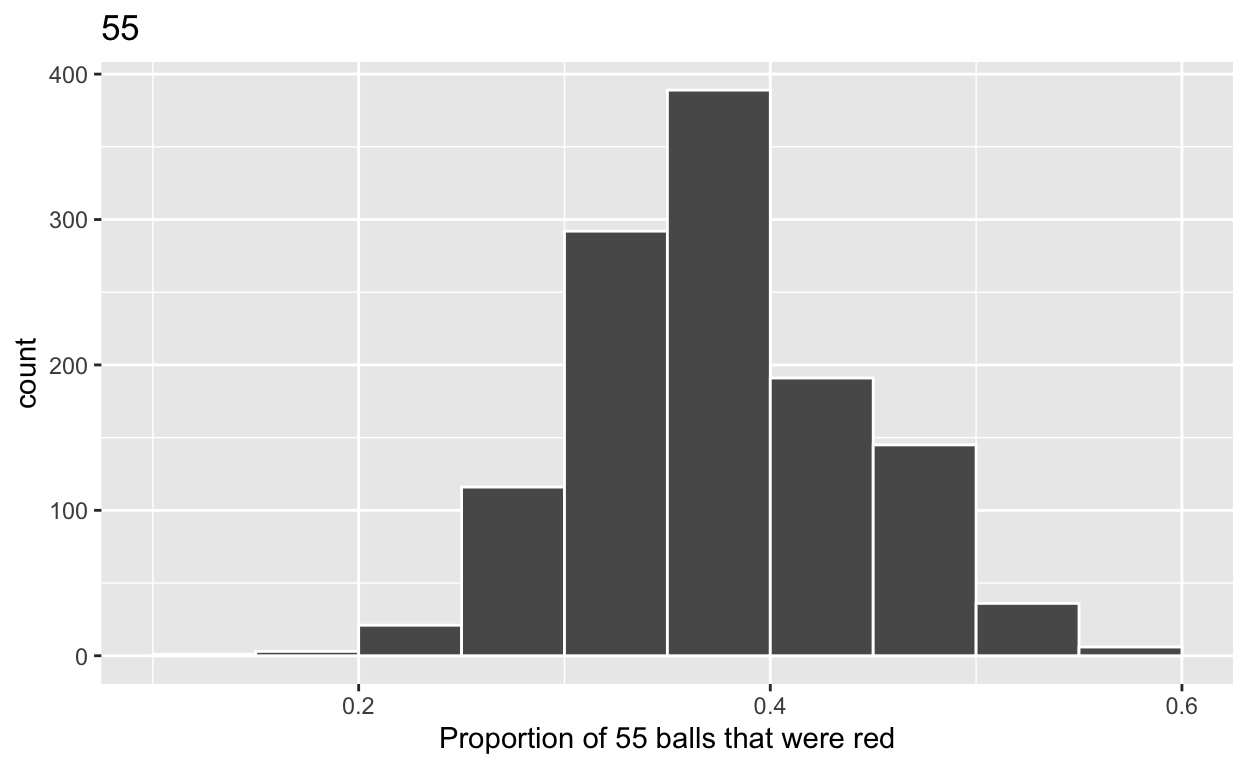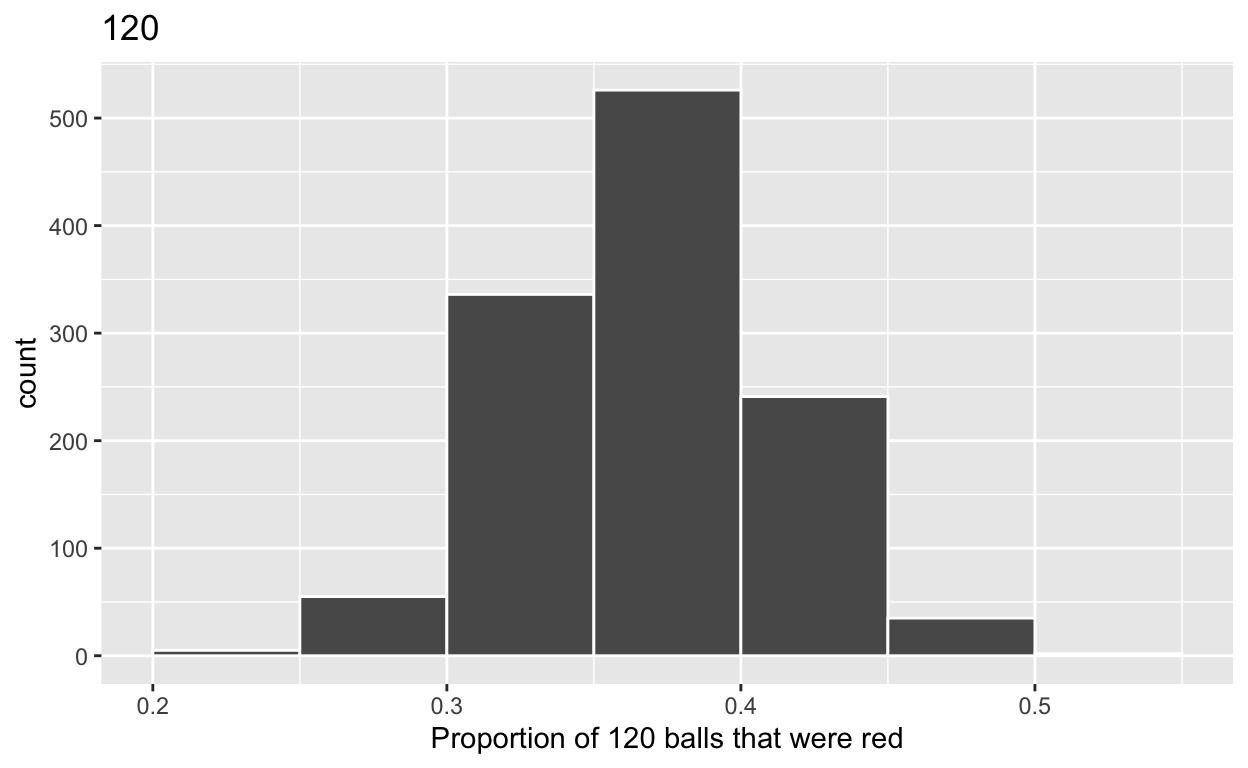# Sampling

Based on Chapter 7 of ModernDive. Code for Quiz 11.

1. Load the R package we will use.
``````library(tidyverse)
library(moderndive)
``````
1. Quiz questions
• Replace all the instances of ‘SEE QUIZ’. These are inputs from your moodle quiz.
• Replace all the instances of ‘???’. These are answers on your moodle quiz.
• Run all the individual code chunks to make sure the answers in this file correspond with your quiz answers
• After you check all your code chunks run then you can knit it. It won’t knit until the ??? are replaced
• The quiz assumes that you have watched the videos and worked through the examples in Chapter 7 of ModernDive

# Question:

7.2.4 in Modern Dive with different sample sizes and repetitions

• Make sure you have installed and loaded the `tidyverse` and the `moderndive` packages
• Fill in the blanks
• Put the command you use in the Rchunks in your Rmd file for this quiz.

Modify the code for comparing different sample sizes from the virtual `bowl`

Segment 1: sample size = 30

1a) Take 1200 samples of size of 30 instead of 1000 replicates of size 25 from the `bowl` dataset. Assign the output to `virtual_samples_30`

``````virtual_samples_30  <- bowl  %>%
rep_sample_n(size = 30, reps = 1200)
``````

1b) Compute resulting 1200 replicates of proportion red

• start with `virtual_samples_30` THEN
• group_by replicate THEN
• create variable red equal to the sum of all the red balls
• create variable prop_red equal to variable red / 30
• assign the output to `virtual_prop_red_30`
``````virtual_prop_red_30 <- virtual_samples_30 %>%
group_by(replicate) %>%
summarize(red = sum(color == "red")) %>%
mutate(prop_red = red / 30)
``````

1c) Plot distribution of `virtual_prop_red_30` via a histogram

Use labs to:

• label x axis = “Proportion of 30 that were red”
• create title = “30”
``````ggplot(virtual_prop_red_30, aes(x = prop_red)) +
geom_histogram(binwidth = 0.05, boundary = 0.4, color = "white") +
labs(x = "Proportion of 30 balls that were red", title = "30")
``````Segment 2: sample size = 55

2a) Take 1200 samples of size of 55 instead of 1000 replicates of size 50. Assign the output to `virtual_samples_55`

``````virtual_samples_55  <- bowl  %>%
rep_sample_n(size = 55, reps = 1200)
``````

2b) Compute resulting 55 replicates of proportion red

start with `virtual_samples_55` THEN group_by replicate THEN create variable red equal to the sum of all the red balls create variable prop_red equal to variable red / 55 Assign the output to `virtual_prop_red_55`

``````virtual_prop_red_55 <- virtual_samples_55 %>%
group_by(replicate) %>%
summarize(red = sum(color == "red")) %>%
mutate(prop_red = red / 55)
``````

2c) Plot distribution of `virtual_prop_red_55` via a histogram use labs to

• label x axis = “Proportion of 55 balls that were red”
• create title = “55”
``````ggplot(virtual_prop_red_55, aes(x = prop_red)) +
geom_histogram(binwidth = 0.05, boundary = 0.4, color = "white") +
labs(x = "Proportion of 55 balls that were red", title = "55")
``````Segment 3: sample size = 120

3a) Take 1200 samples of size of 120 instead of 1000 replicates of size 50. Assign the output to `virtual_samples_120`

``````virtual_samples_120  <- bowl  %>%
rep_sample_n(size = 120, reps = 1200)
``````

3b) Compute resulting 1200 replicates of proportion red

• start with `virtual_samples_120` THEN
• group_by replicate THEN
• create variable red equal to the sum of all the red balls
• create variable prop_red equal to variable red / 120
• assign the output to `virtual_prop_red_120`
``````virtual_prop_red_120 <- virtual_samples_120 %>%
group_by(replicate) %>%
summarize(red = sum(color == "red")) %>%
mutate(prop_red = red / 120)
``````

3c) Plot distribution of `virtual_prop_red_120` via a histogram

Use labs to:

• label x axis = “Proportion of 120 balls that were red”
• create title = “120”
``````ggplot(virtual_prop_red_120, aes(x = prop_red)) +
geom_histogram(binwidth = 0.05, boundary = 0.4, color = "white") +
labs(x = "Proportion of 120 balls that were red", title = "120")
````````````ggsave(filename = "preview.png",
path = here::here("_posts", "2022-04-19-sampling"))
``````

Calculate the standard deviations for your three sets of 1200 values of `prop_red` using the `standard deviation`

n = 30

``````virtual_prop_red_30 %>%
summarize(sd = sd(prop_red))
``````
``````# A tibble: 1 × 1
sd
<dbl>
1 0.0890``````

n = 55

``````virtual_prop_red_55 %>%
summarize(sd = sd(prop_red))
``````
``````# A tibble: 1 × 1
sd
<dbl>
1 0.0657``````

n = S120

``````virtual_prop_red_120 %>%
summarize(sd = sd(prop_red))
``````
``````# A tibble: 1 × 1
sd
<dbl>
1 0.0429``````

The distribution with sample size, n = 120, has the smallest standard deviation (spread) around the estimated proportion of red balls.